Скачать презентацию Chapter Four The Meaning of Interest Rates Future

83caf2caca613cc8c72a34125e2d1b61.ppt

• Количество слайдов: 38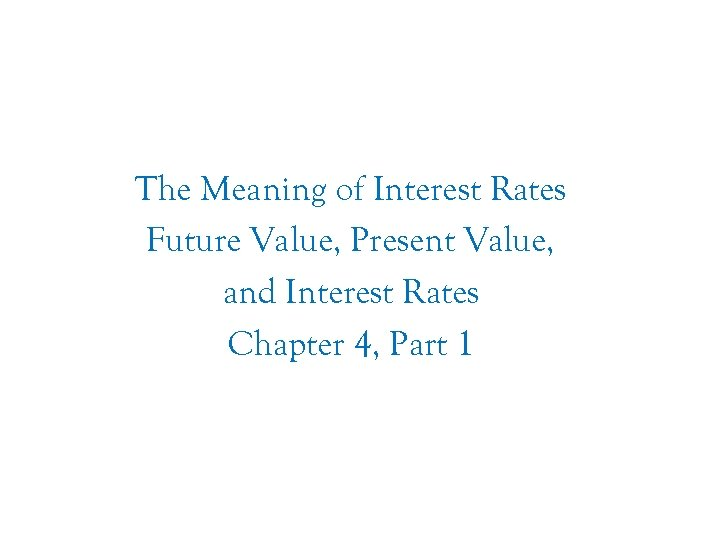Chapter Four The Meaning of Interest Rates Future Value, Present Value, and Interest Rates Chapter 4, Part 1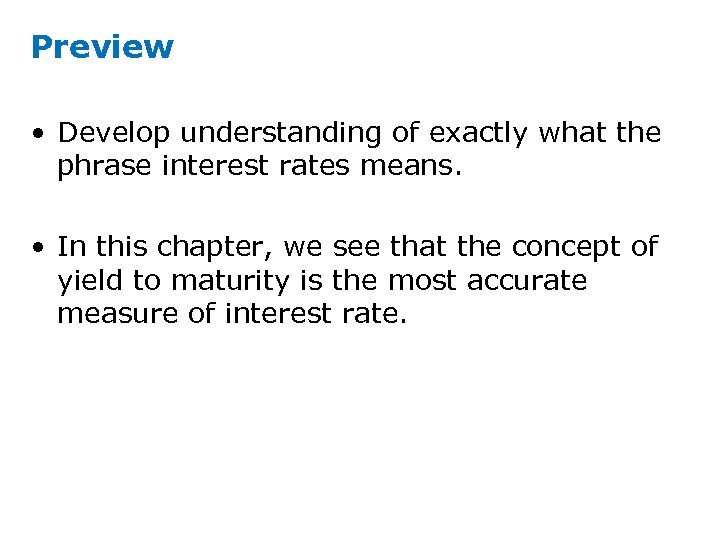Preview • Develop understanding of exactly what the phrase interest rates means. • In this chapter, we see that the concept of yield to maturity is the most accurate measure of interest rate.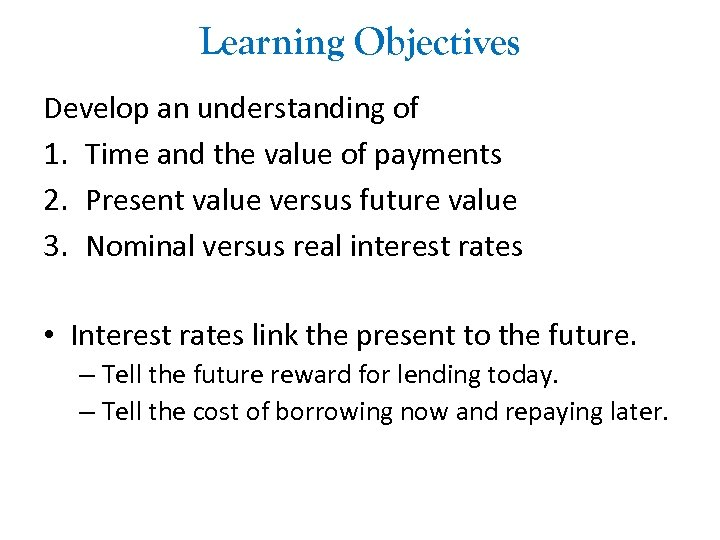Learning Objectives Develop an understanding of 1. Time and the value of payments 2. Present value versus future value 3. Nominal versus real interest rates • Interest rates link the present to the future. – Tell the future reward for lending today. – Tell the cost of borrowing now and repaying later.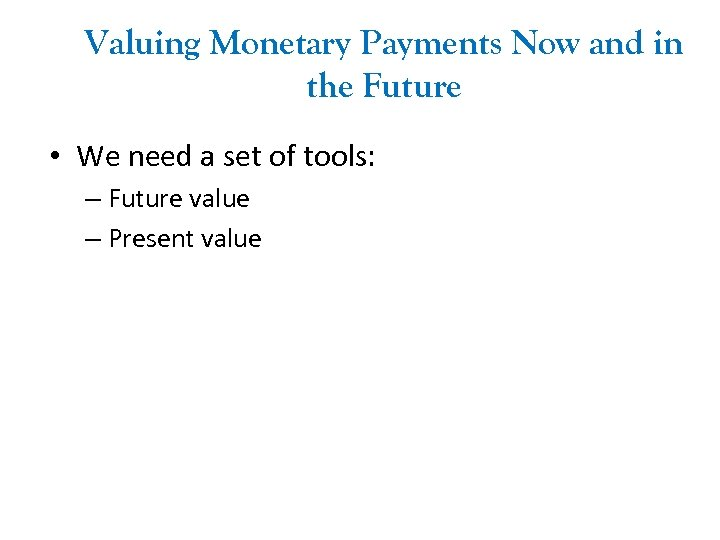Valuing Monetary Payments Now and in the Future • We need a set of tools: – Future value – Present value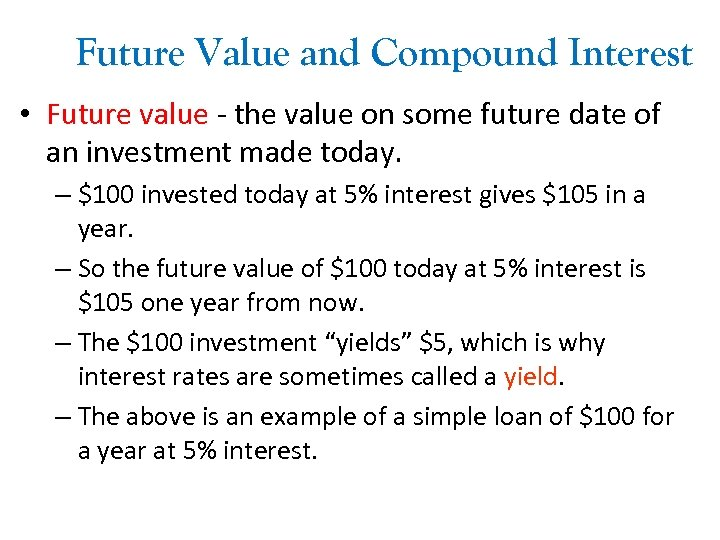Future Value and Compound Interest • Future value - the value on some future date of an investment made today. – \$100 invested today at 5% interest gives \$105 in a year. – So the future value of \$100 today at 5% interest is \$105 one year from now. – The \$100 investment “yields” \$5, which is why interest rates are sometimes called a yield. – The above is an example of a simple loan of \$100 for a year at 5% interest.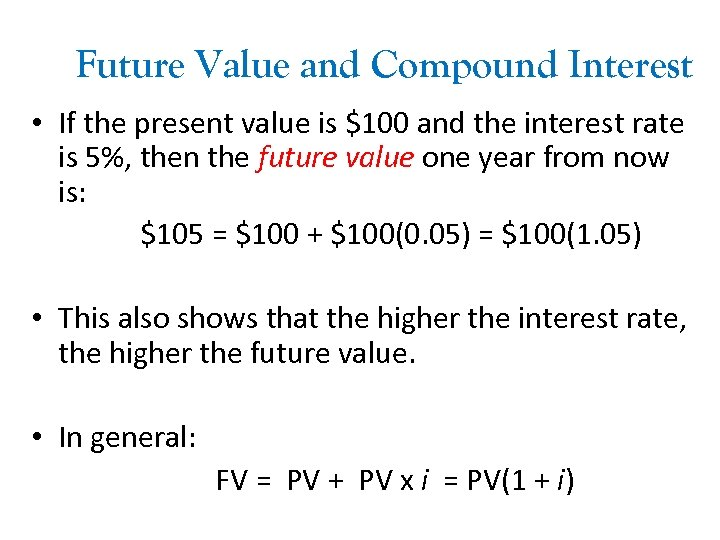Future Value and Compound Interest • If the present value is \$100 and the interest rate is 5%, then the future value one year from now is: \$105 = \$100 + \$100(0. 05) = \$100(1. 05) • This also shows that the higher the interest rate, the higher the future value. • In general: FV = PV + PV x i = PV(1 + i)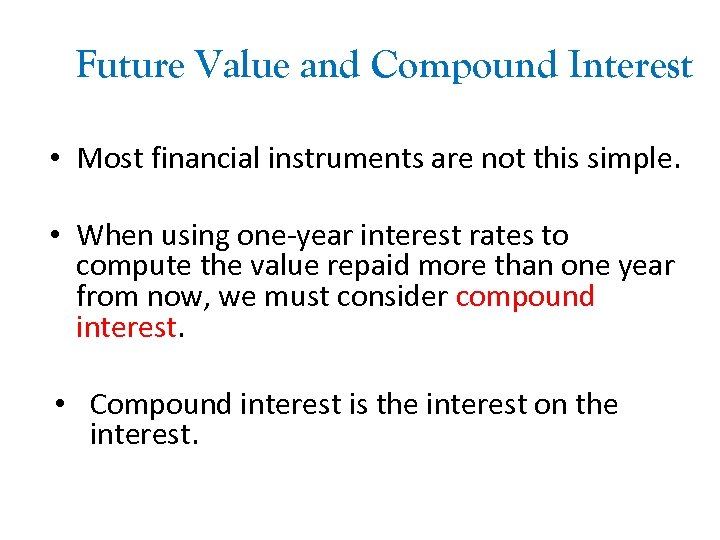Future Value and Compound Interest • Most financial instruments are not this simple. • When using one-year interest rates to compute the value repaid more than one year from now, we must consider compound interest. • Compound interest is the interest on the interest.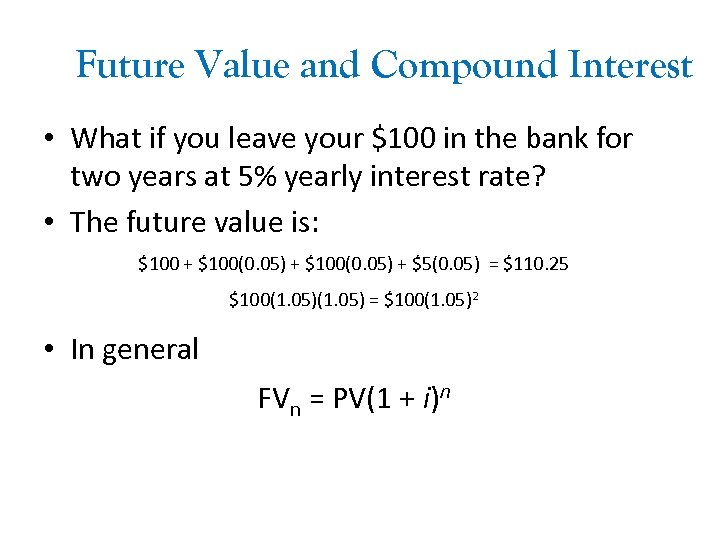Future Value and Compound Interest • What if you leave your \$100 in the bank for two years at 5% yearly interest rate? • The future value is: \$100 + \$100(0. 05) + \$5(0. 05) = \$110. 25 \$100(1. 05) = \$100(1. 05)2 • In general FVn = PV(1 + i)n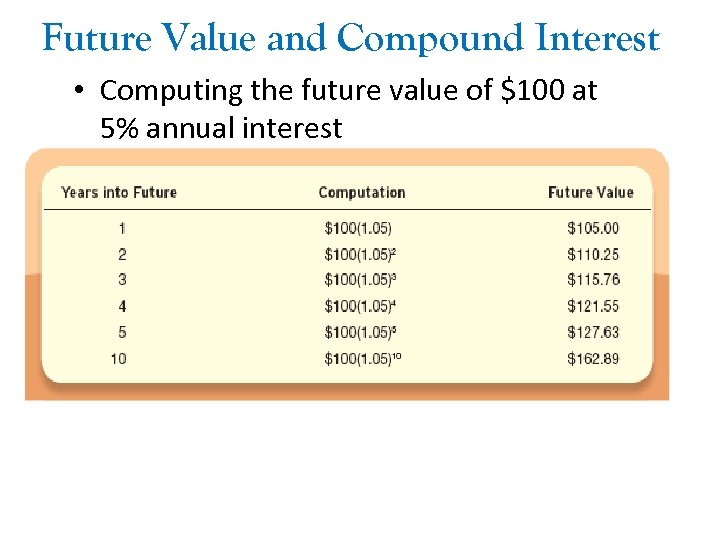Future Value and Compound Interest • Computing the future value of \$100 at 5% annual interest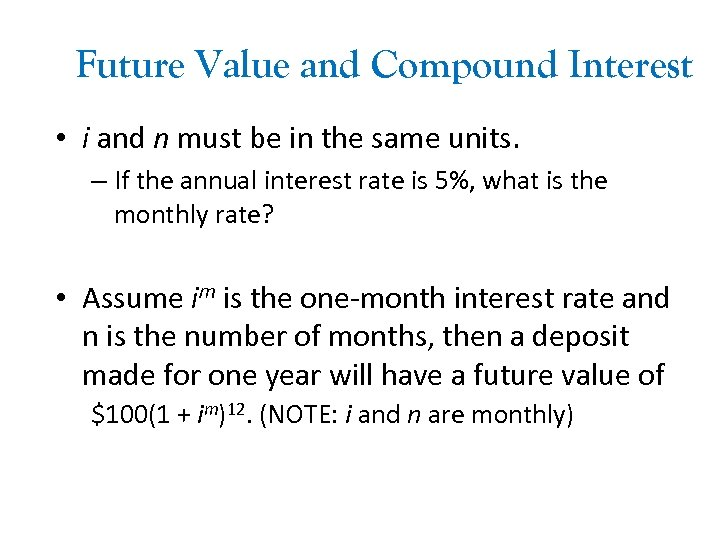Future Value and Compound Interest • i and n must be in the same units. – If the annual interest rate is 5%, what is the monthly rate? • Assume im is the one-month interest rate and n is the number of months, then a deposit made for one year will have a future value of \$100(1 + im)12. (NOTE: i and n are monthly)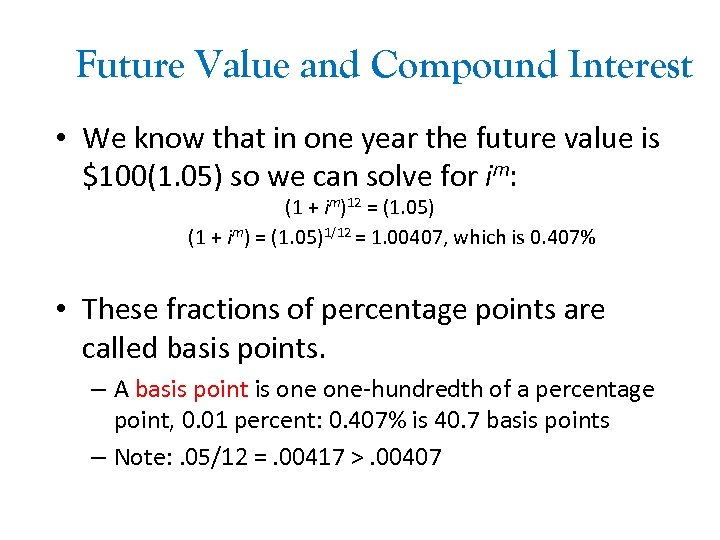Future Value and Compound Interest • We know that in one year the future value is \$100(1. 05) so we can solve for im: (1 + im)12 = (1. 05) (1 + im) = (1. 05)1/12 = 1. 00407, which is 0. 407% • These fractions of percentage points are called basis points. – A basis point is one-hundredth of a percentage point, 0. 01 percent: 0. 407% is 40. 7 basis points – Note: . 05/12 =. 00417 >. 00407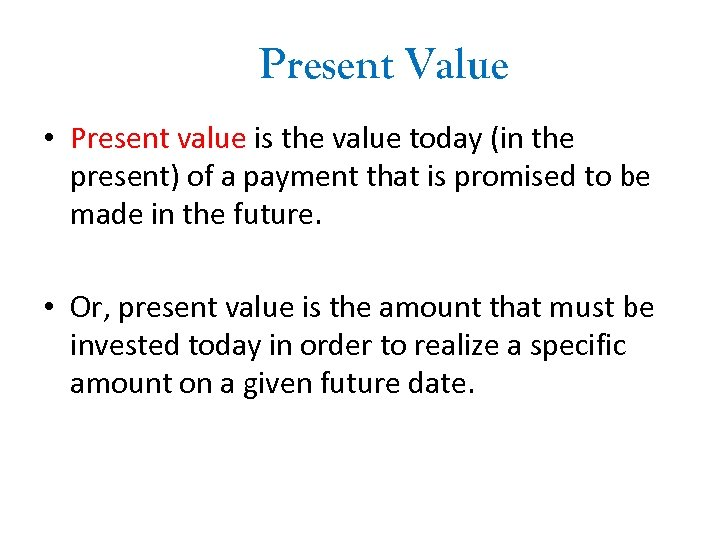Present Value • Present value is the value today (in the present) of a payment that is promised to be made in the future. • Or, present value is the amount that must be invested today in order to realize a specific amount on a given future date.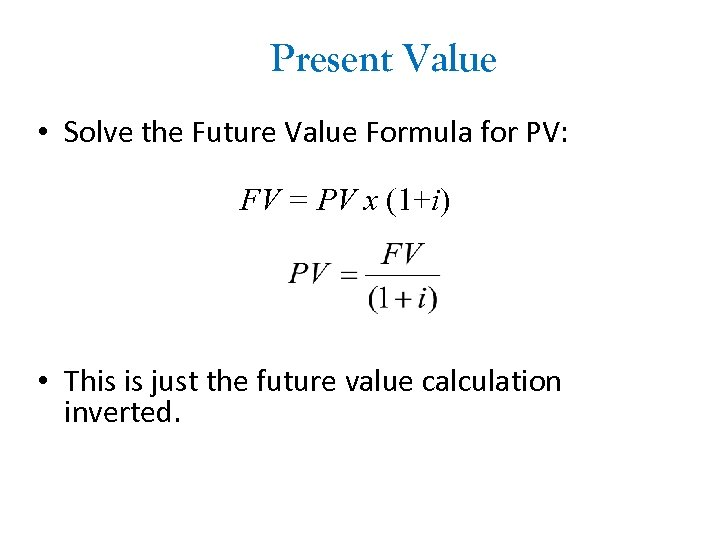Present Value • Solve the Future Value Formula for PV: FV = PV x (1+i) • This is just the future value calculation inverted.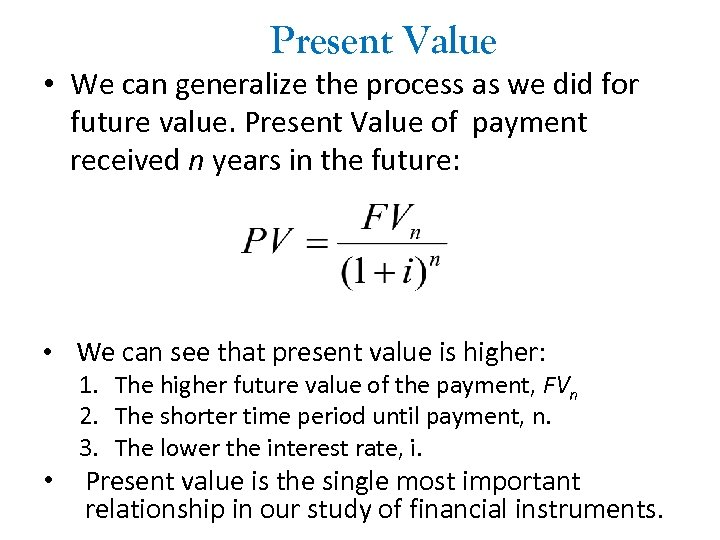Present Value • We can generalize the process as we did for future value. Present Value of payment received n years in the future: • We can see that present value is higher: 1. The higher future value of the payment, FVn 2. The shorter time period until payment, n. 3. The lower the interest rate, i. • Present value is the single most important relationship in our study of financial instruments.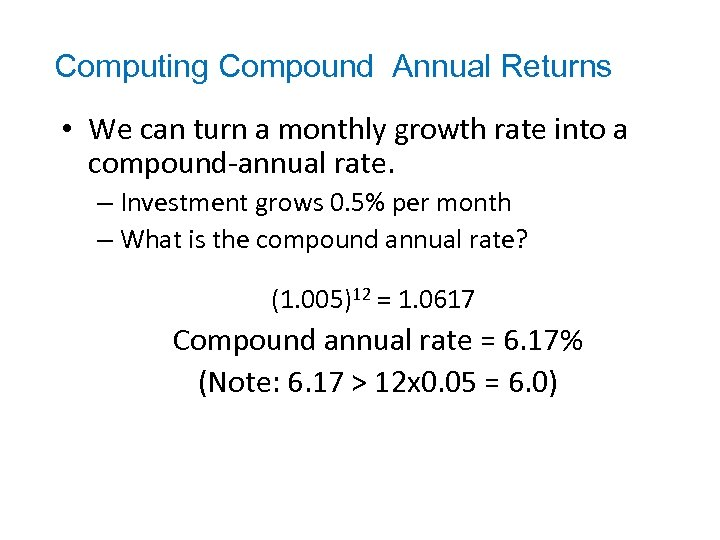Computing Compound Annual Returns • We can turn a monthly growth rate into a compound-annual rate. – Investment grows 0. 5% per month – What is the compound annual rate? (1. 005)12 = 1. 0617 Compound annual rate = 6. 17% (Note: 6. 17 > 12 x 0. 05 = 6. 0)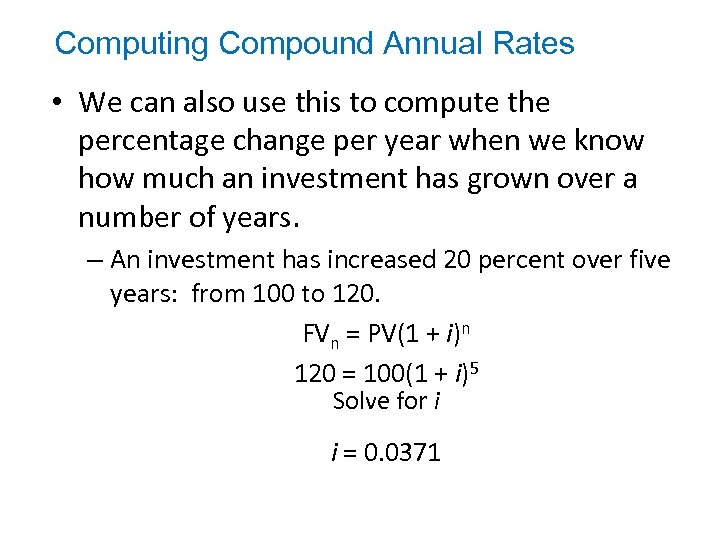Computing Compound Annual Rates • We can also use this to compute the percentage change per year when we know how much an investment has grown over a number of years. – An investment has increased 20 percent over five years: from 100 to 120. FVn = PV(1 + i)n 120 = 100(1 + i)5 Solve for i i = 0. 0371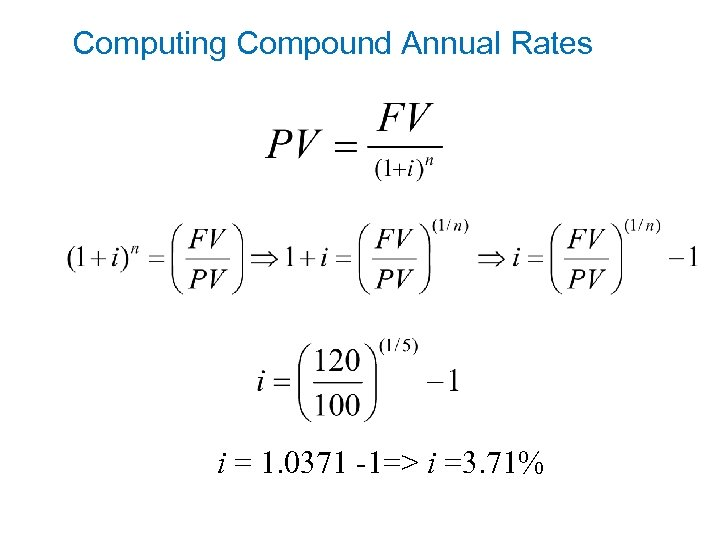Computing Compound Annual Rates i = 1. 0371 -1=> i =3. 71%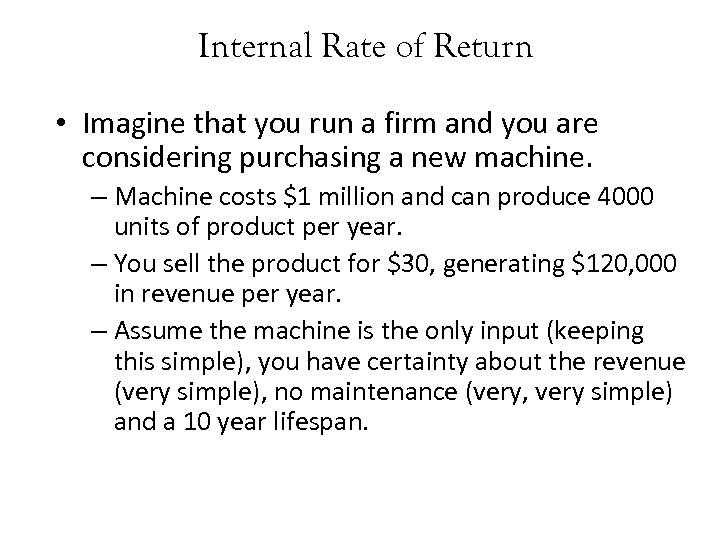Internal Rate of Return • Imagine that you run a firm and you are considering purchasing a new machine. – Machine costs \$1 million and can produce 4000 units of product per year. – You sell the product for \$30, generating \$120, 000 in revenue per year. – Assume the machine is the only input (keeping this simple), you have certainty about the revenue (very simple), no maintenance (very, very simple) and a 10 year lifespan.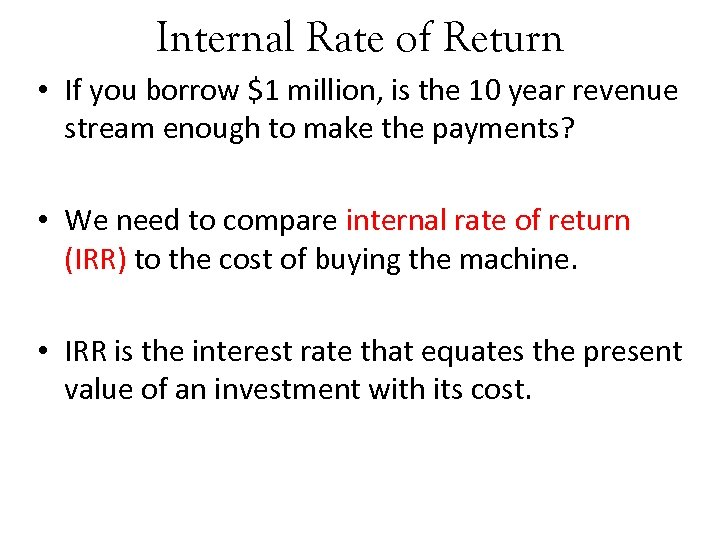Internal Rate of Return • If you borrow \$1 million, is the 10 year revenue stream enough to make the payments? • We need to compare internal rate of return (IRR) to the cost of buying the machine. • IRR is the interest rate that equates the present value of an investment with its cost.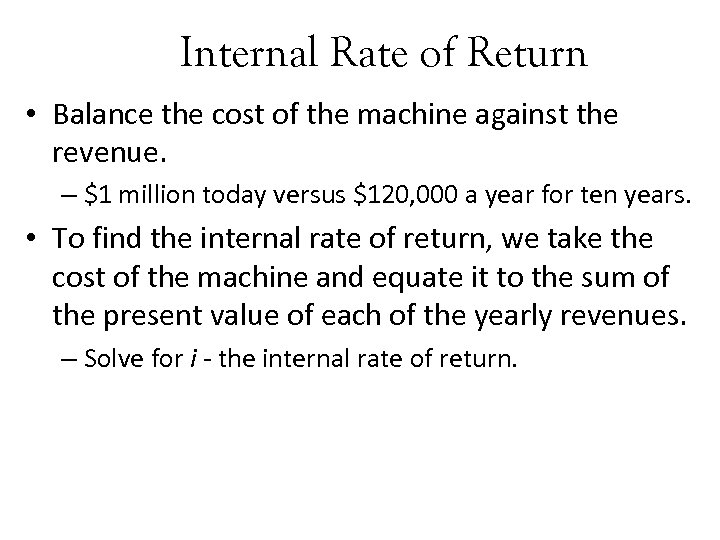Internal Rate of Return • Balance the cost of the machine against the revenue. – \$1 million today versus \$120, 000 a year for ten years. • To find the internal rate of return, we take the cost of the machine and equate it to the sum of the present value of each of the yearly revenues. – Solve for i - the internal rate of return.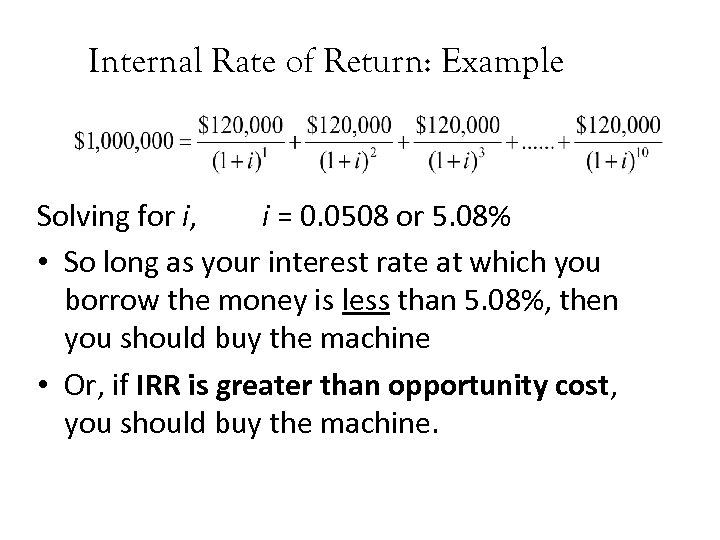Internal Rate of Return: Example Solving for i, i = 0. 0508 or 5. 08% • So long as your interest rate at which you borrow the money is less than 5. 08%, then you should buy the machine • Or, if IRR is greater than opportunity cost, you should buy the machine.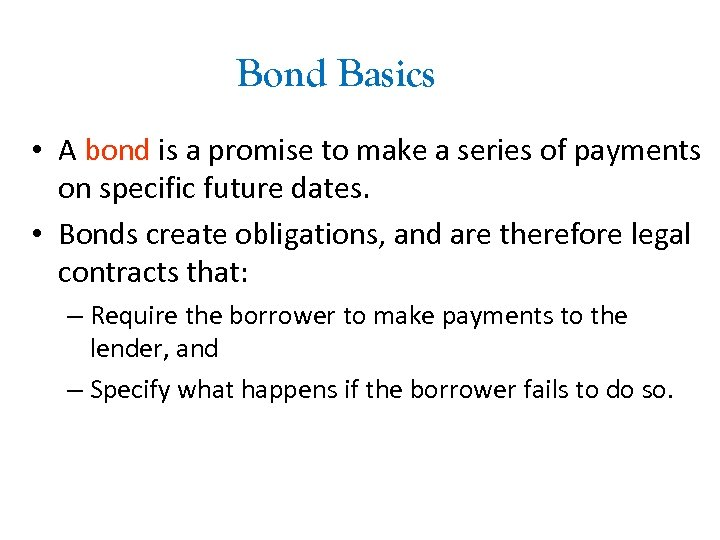Bond Basics • A bond is a promise to make a series of payments on specific future dates. • Bonds create obligations, and are therefore legal contracts that: – Require the borrower to make payments to the lender, and – Specify what happens if the borrower fails to do so.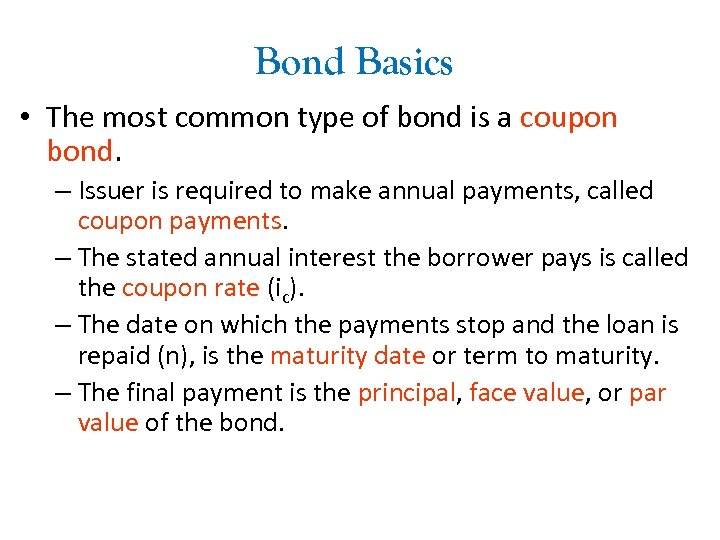Bond Basics • The most common type of bond is a coupon bond. – Issuer is required to make annual payments, called coupon payments. – The stated annual interest the borrower pays is called the coupon rate (ic). – The date on which the payments stop and the loan is repaid (n), is the maturity date or term to maturity. – The final payment is the principal, face value, or par value of the bond.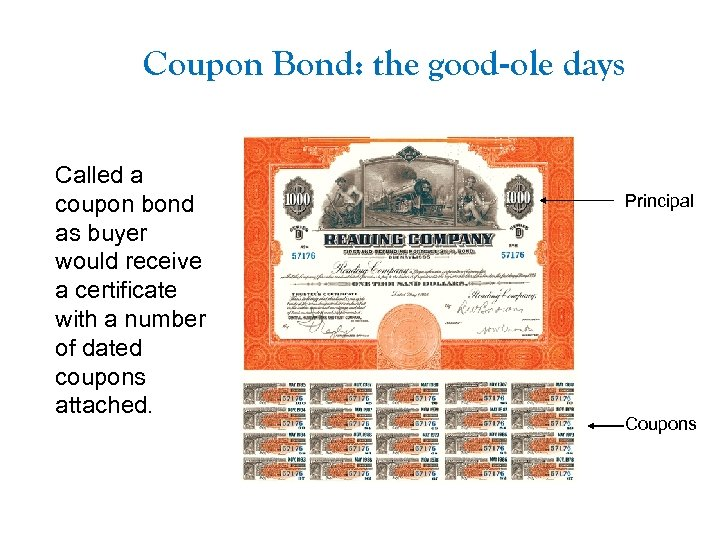Coupon Bond: the good-ole days Called a coupon bond as buyer would receive a certificate with a number of dated coupons attached. Principal Coupons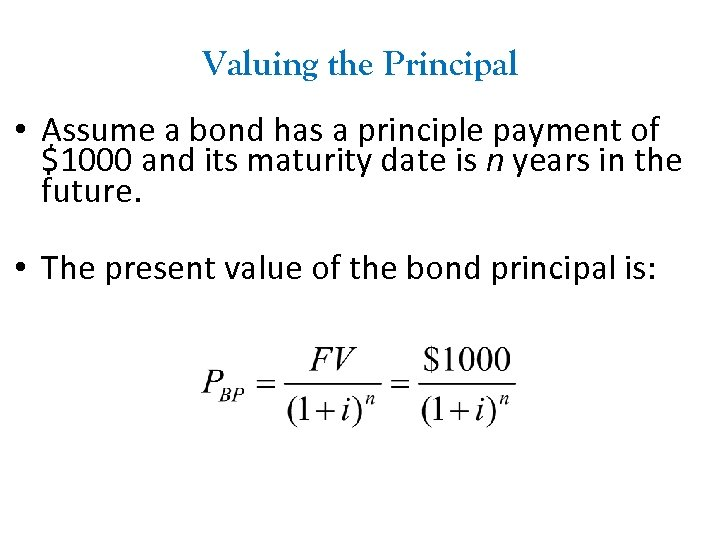Valuing the Principal • Assume a bond has a principle payment of \$1000 and its maturity date is n years in the future. • The present value of the bond principal is: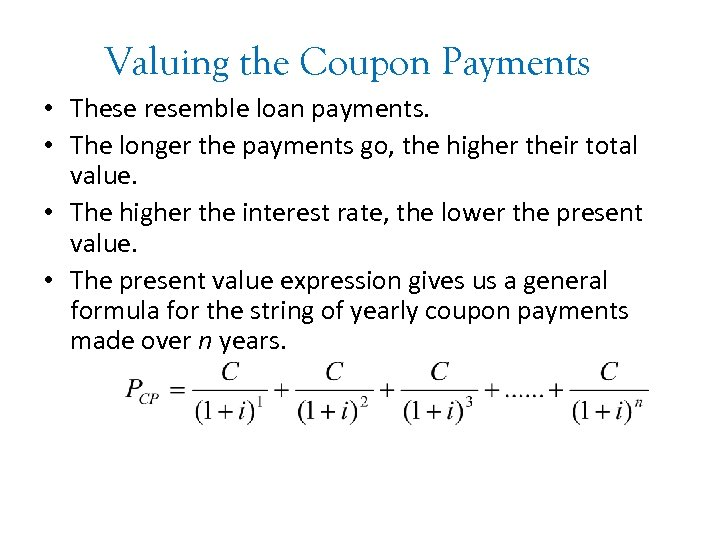Valuing the Coupon Payments • These resemble loan payments. • The longer the payments go, the higher their total value. • The higher the interest rate, the lower the present value. • The present value expression gives us a general formula for the string of yearly coupon payments made over n years.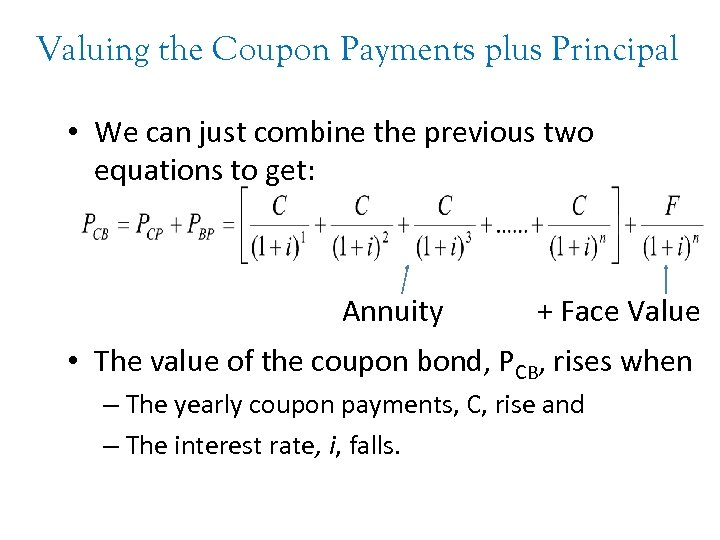Valuing the Coupon Payments plus Principal • We can just combine the previous two equations to get: Annuity + Face Value • The value of the coupon bond, PCB, rises when – The yearly coupon payments, C, rise and – The interest rate, i, falls.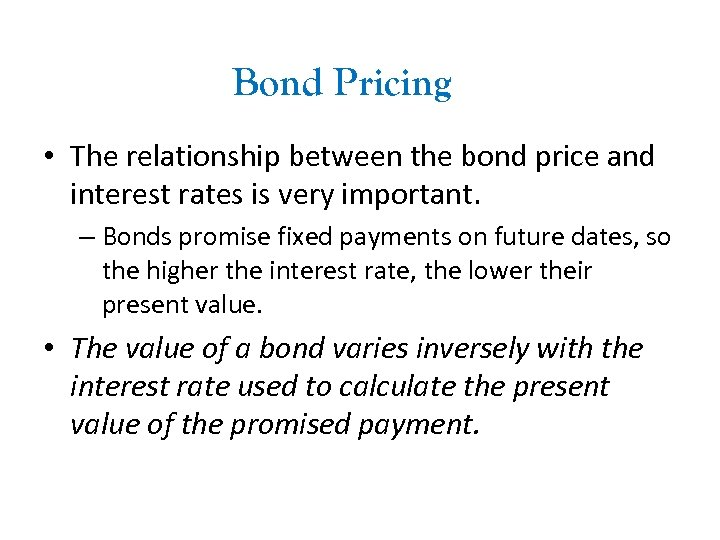Bond Pricing • The relationship between the bond price and interest rates is very important. – Bonds promise fixed payments on future dates, so the higher the interest rate, the lower their present value. • The value of a bond varies inversely with the interest rate used to calculate the present value of the promised payment.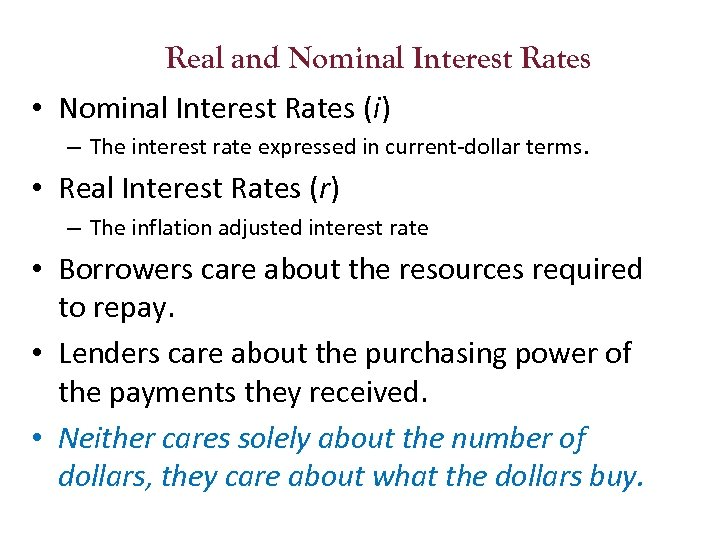Real and Nominal Interest Rates • Nominal Interest Rates (i) – The interest rate expressed in current-dollar terms. • Real Interest Rates (r) – The inflation adjusted interest rate • Borrowers care about the resources required to repay. • Lenders care about the purchasing power of the payments they received. • Neither cares solely about the number of dollars, they care about what the dollars buy.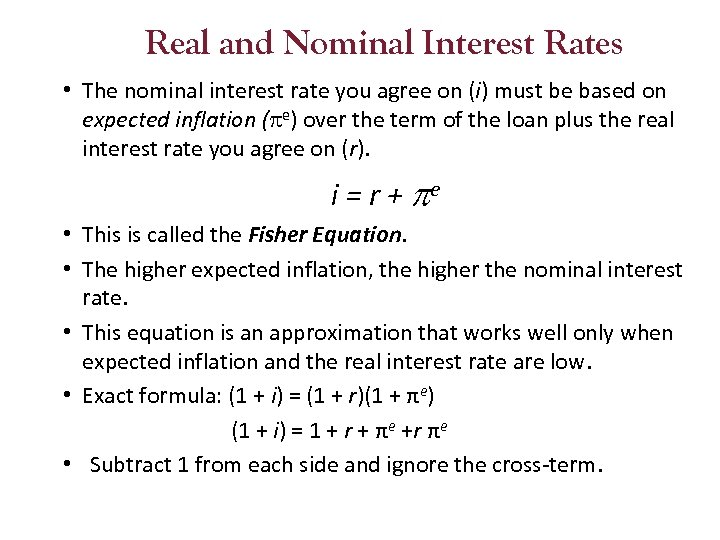Real and Nominal Interest Rates • The nominal interest rate you agree on (i) must be based on expected inflation ( e) over the term of the loan plus the real interest rate you agree on (r). i = r + e • This is called the Fisher Equation. • The higher expected inflation, the higher the nominal interest rate. • This equation is an approximation that works well only when expected inflation and the real interest rate are low. • Exact formula: (1 + i) = (1 + r)(1 + πe) (1 + i) = 1 + r + πe +r πe • Subtract 1 from each side and ignore the cross-term.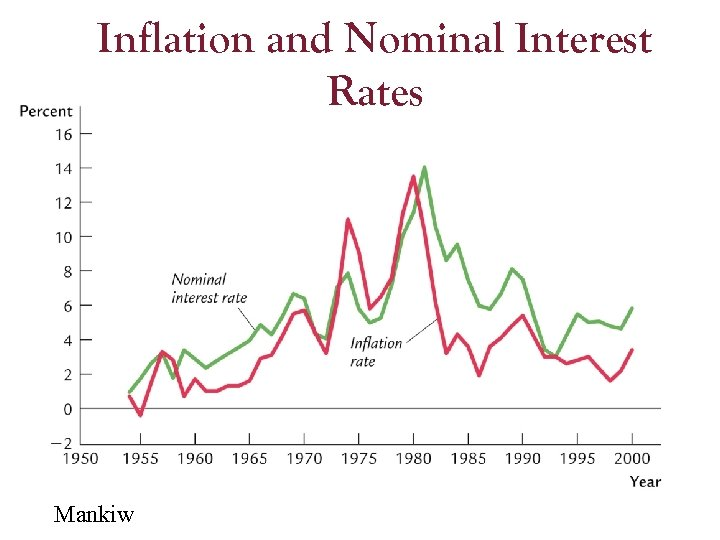Inflation and Nominal Interest Rates Mankiw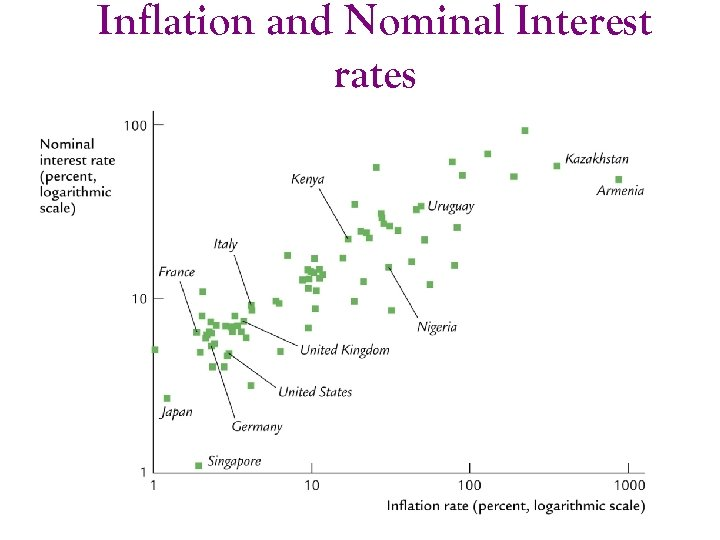Inflation and Nominal Interest rates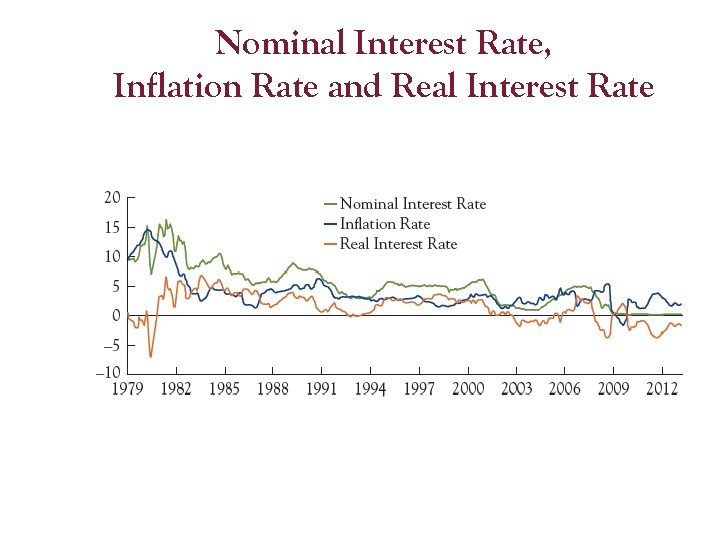Nominal Interest Rate, Inflation Rate and Real Interest Rate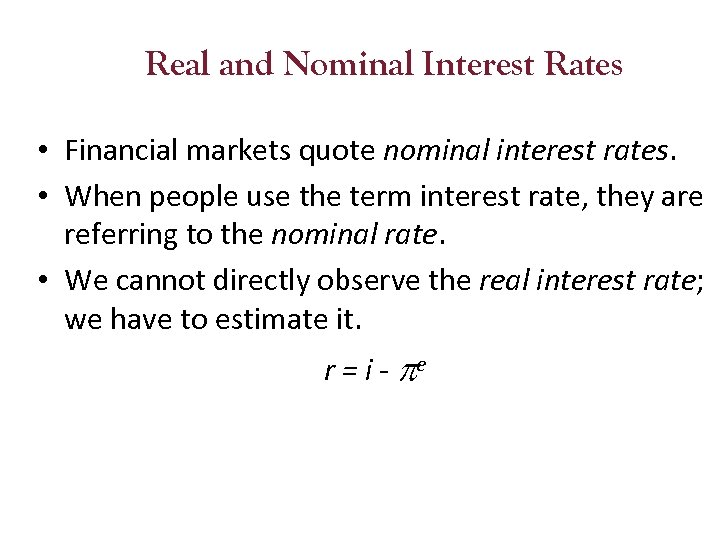Real and Nominal Interest Rates • Financial markets quote nominal interest rates. • When people use the term interest rate, they are referring to the nominal rate. • We cannot directly observe the real interest rate; we have to estimate it. r = i - e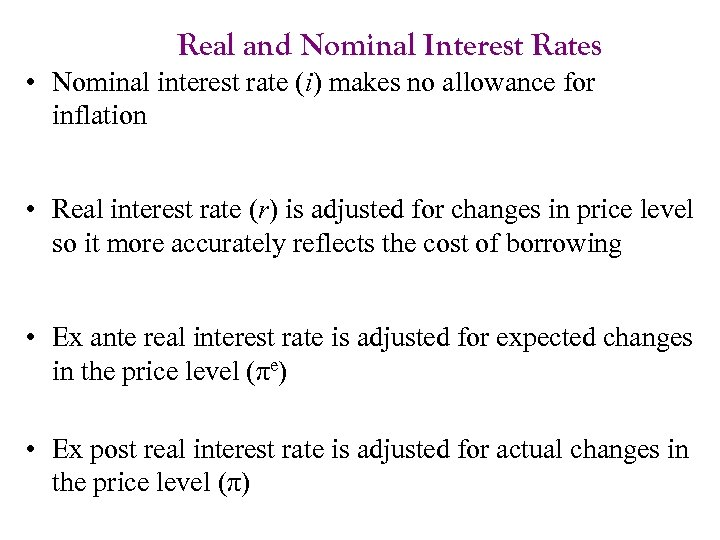Real and Nominal Interest Rates • Nominal interest rate (i) makes no allowance for inflation • Real interest rate (r) is adjusted for changes in price level so it more accurately reflects the cost of borrowing • Ex ante real interest rate is adjusted for expected changes in the price level (πe) • Ex post real interest rate is adjusted for actual changes in the price level (π)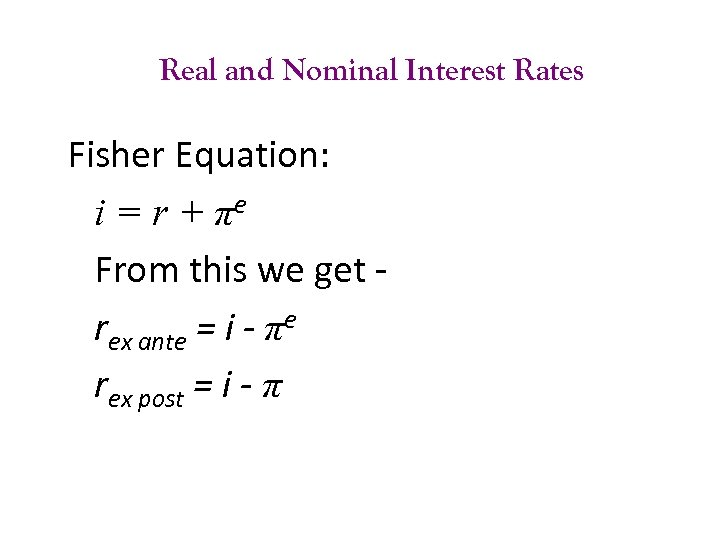Real and Nominal Interest Rates Fisher Equation: i = r + πe From this we get rex ante = i - πe rex post = i - π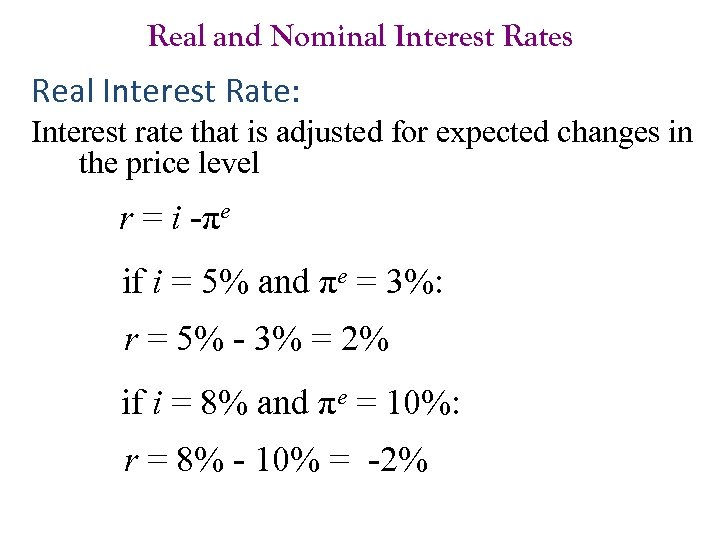Real and Nominal Interest Rates Real Interest Rate: Interest rate that is adjusted for expected changes in the price level r = i -πe if i = 5% and πe = 3%: r = 5% - 3% = 2% if i = 8% and πe = 10%: r = 8% - 10% = -2%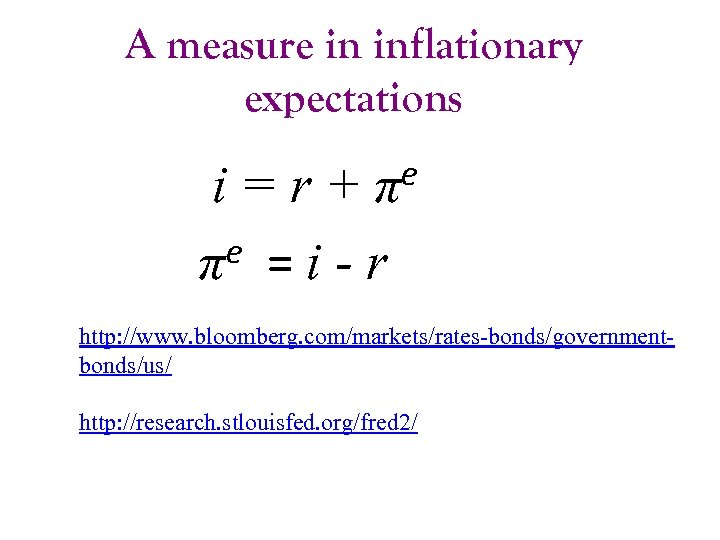A measure in inflationary expectations e π i=r+ e =i-r π http: //www. bloomberg. com/markets/rates-bonds/governmentbonds/us/ http: //research. stlouisfed. org/fred 2/